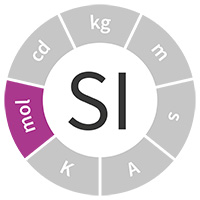Close
Close
SI units

# mole (mol)

The mole is the SI unit of amount of substanceThe mole is the SI unit used to express chemical amount. Accurate chemical measurements are vital for a range of applications including accurately administering medicines, monitoring environmental pollution, assuring food and water quality and optimising chemical engineering processes.

## Definition

One mole contains exactly 6.022 140 76 × 1023 elementary entities. This number is the fixed numerical value of the Avogadro constant, 𝑁A, when expressed in the unit mol-1 and is called the Avogadro number.

The amount of substance, symbol 𝑛, of a system is a measure of the number of specified elementary entities. An elementary entity may be an atom, a molecule, an ion, an electron, any other particle or specified group of particles.

This was a new definition in May 2019.

Application

Atoms and molecules don’t react together in terms of mass-based relationships, but instead in terms of amount of substance‑based relationships. We often call this stoichiometry and this is why the presence of the mole within the SI is useful.

## Did you know?

• If an atom was the size of a grapefruit, then an Avogadro number of them would have approximately the same volume as the Earth
• The mole is used to quantify atoms or molecules that can chemically react on a one-to-one (or simple whole number) basis, as this is much more useful than measuring their mass or volume
• We don’t use the mole to describe things that aren’t fundamental entities like atoms, ions and molecules, so it is not just a large number that can be used for, say, a mole of stars

The science behind the unit

The mole is used to describe practical-sized quantities of material in the macroscopic world. The Avogadro constant provides the link between this macroscopic world and the microscopic world of atoms and molecules. As a result of the definition, the mole contains a defined number of entities, usually atoms or molecules. This number is the numercial value of the Avogadro constant (𝑁A). (It would take you twenty thousand million million years to count up to this number if you were counting at one number per second).

The mole can be realised by certain very well controlled experimental methods known as 'primary methods'. One example is by weighing a chemical of known purity.

Find out about NPL's research in this area